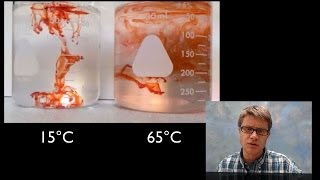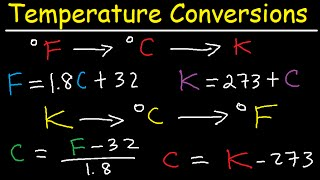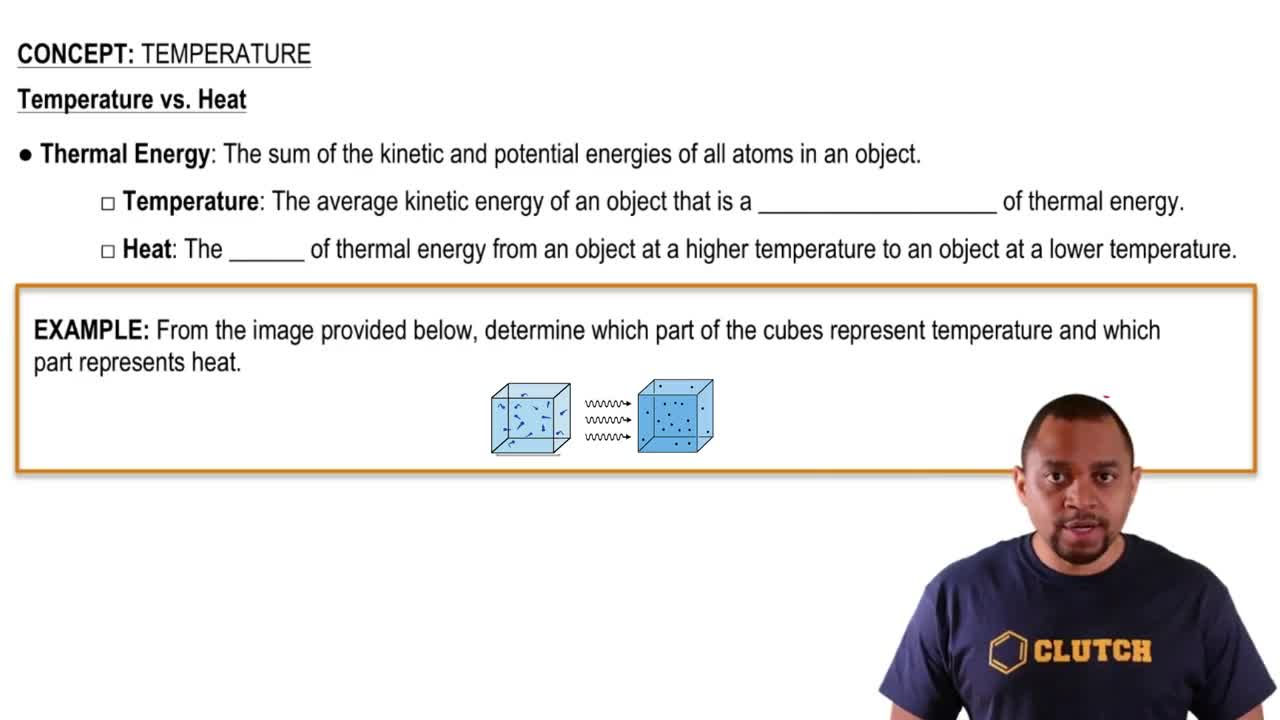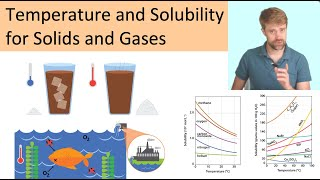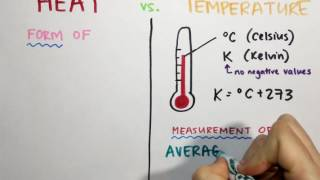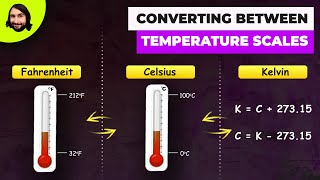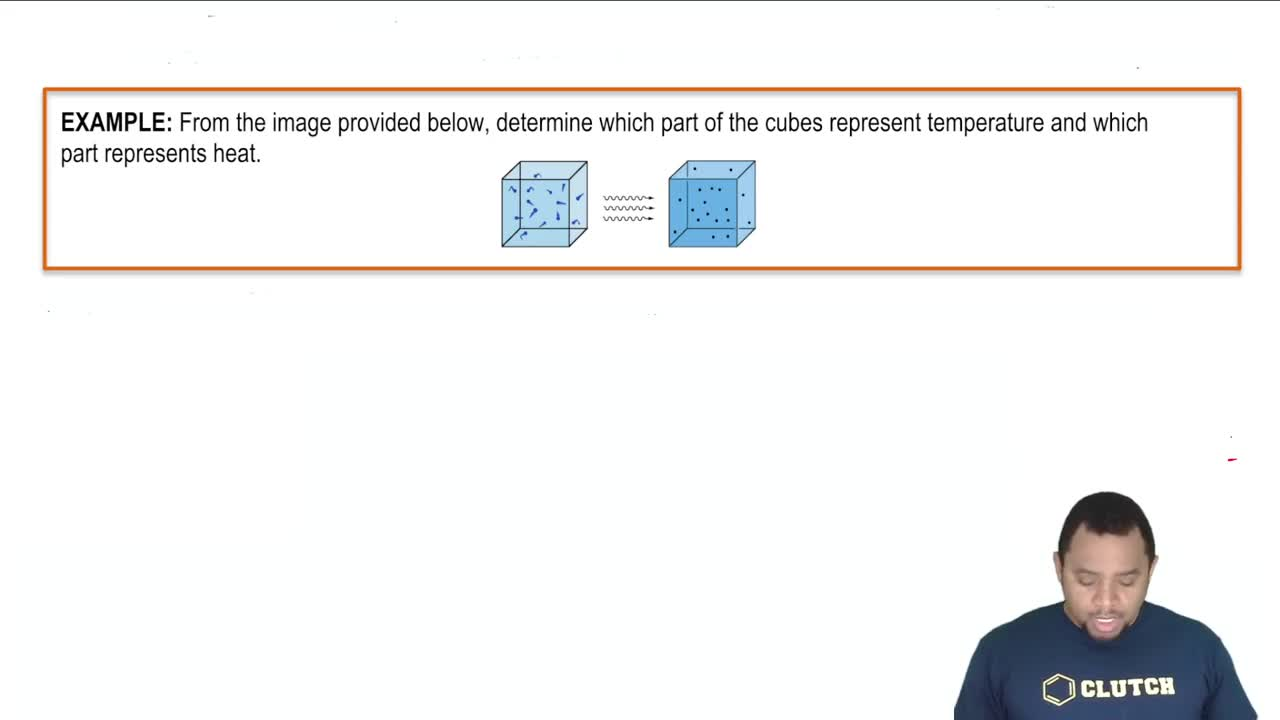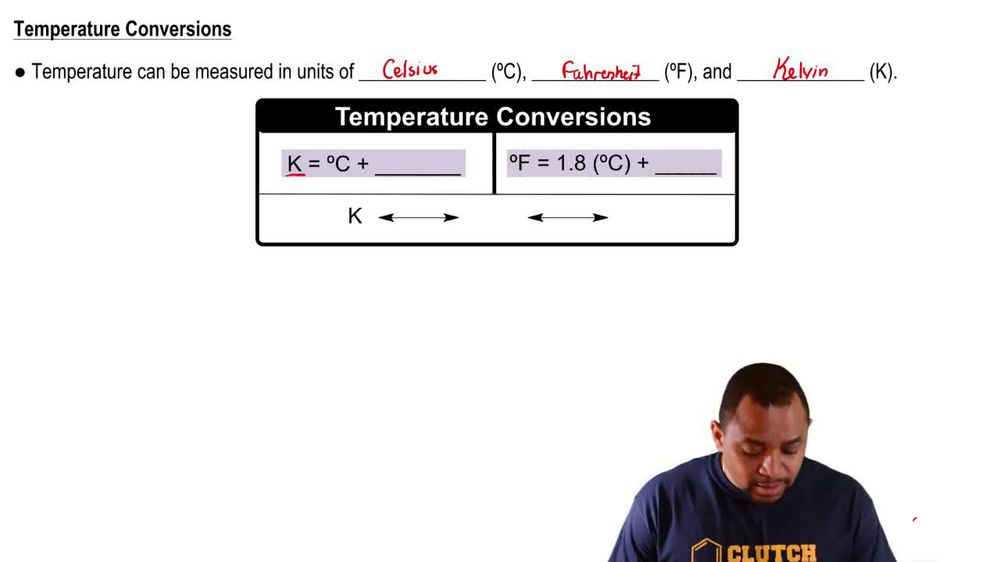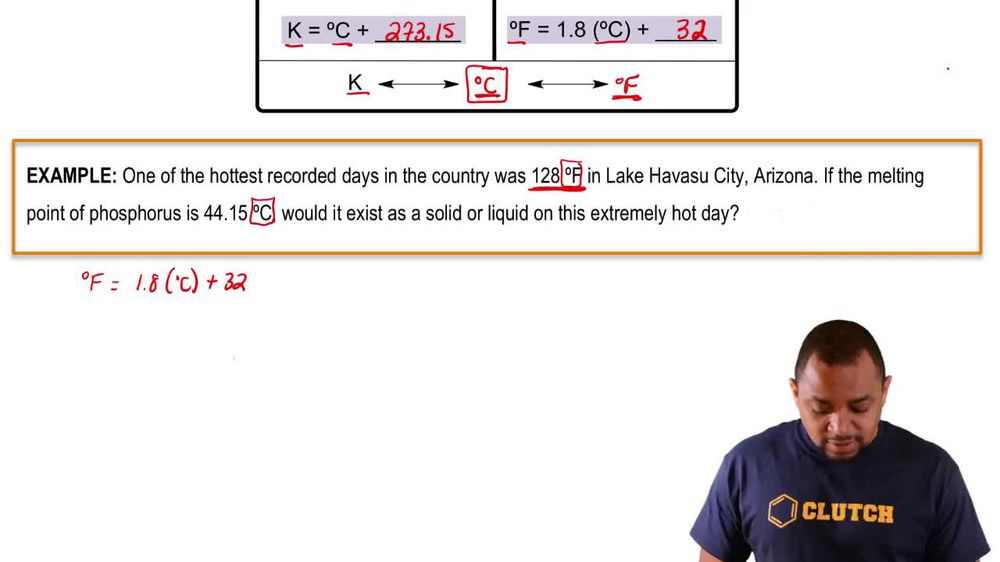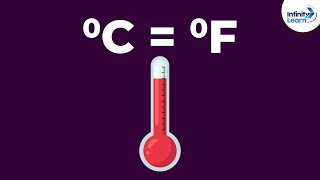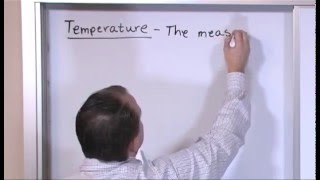Start typing, then use the up and down arrows to select an option from the list.
1. 1. Intro to General Chemistry2. Temperature
Problem

# At a certain point, the Celsius and Fahrenheit scales “cross,” giving the same numerical value on both. At what tempera-ture does this crossover occur?

Relevant Solution3m
Play a video:
Hi everyone. This problem reads the Celsius and Fahrenheit scales cross at a point where their numerical values are the same. What is the temperature at which the crossover happens? Okay, so this is what we want to figure out the temperature at which the crossover happens. So let's go ahead and write our equation for the two scales where they relate to each other and that equation is degrees Fahrenheit is equal to degrees Celsius times 9/5 plus 32. So the question is asking us for the point where the numerical values are the same. So this is where the crossover happens. So what that means is at the crossover degrees Fahrenheit is going to equal degrees Celsius and we want to know what is that value when they both equal each other. Okay, and so what we can do is we can set our equation, we can set it equal to each other, we can choose one of them to set equal to each other. So let's go ahead and choose degrees Fahrenheit. So wherever we see degrees Celsius, we're going to set that equal two F. And what that looks like when we write it out is degrees Fahrenheit is going to equal degrees Fahrenheit times 9/ plus 32. Okay, so we just replaced our degrees Celsius with degrees Fahrenheit, we set it equal to each other. So now what we want to do is we want to solve for degrees Fahrenheit. So let's go ahead and start off by bringing degrees Fahrenheit to one side. Alright, so what that's gonna look like when we bring degrees Fahrenheit to one side is we're going to have negative 32 is equal to negative degrees Fahrenheit times 9/5 minus degrees Fahrenheit. So see here we have our degrees Fahrenheit on one side of our equation. Now that we have it on one side of our equation, we're going to go ahead and take our minus degrees Fahrenheit and that's going to become minus one. Ok, so negative 32 equals negative degrees Fahrenheit times 9/5 minus one. Okay, so we can simplify this to be negative, 32 degrees equals negative degrees Fahrenheit, 9/5 minus one is 4/5. Okay, and now what we want to do is isolate our degrees Fahrenheit. Okay, so let's bring it over to the left side degrees. Fahrenheit is going to equal negative 32. Now we're going to flip the fraction because we're bringing it to the other side. So negative 32 times 5/4. So now we can go ahead and simplify this out to solve for degrees Fahrenheit and the value we get is negative 40. So our answer for this problem is that negative 40 F Which is also negative 40°C is where the crossover is going to happen. This is the temperature at which they're both going to equal or where the this is going to be the point at where the numerical values are the same. Okay, so that's it for this problem. I hope this was helpful.